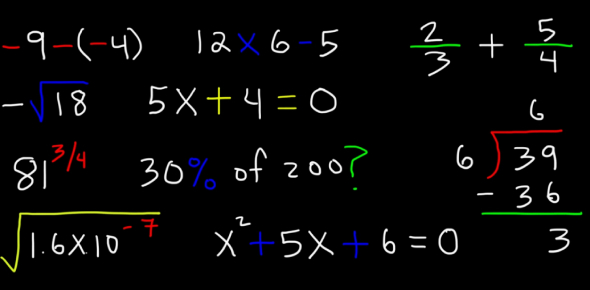# Quiz: Test Your Basic Maths Knowledge!

15 Questions | Total Attempts: 13028SettingsTime: 30 Minute

• 1.
• A.

A rational number

• B.

A natural number

• C.

An irrational number

• D.

Equal to zero

• 2.
If  be a rational number. Then  has decimal expansion which terminates:
• A.

After two decimal places

• B.

After five decimal places

• C.

After three decimal places

• D.

After four decimal places

• 3.
The decimal expansion of  is:
• A.

Terminating

• B.

Non-terminating

• C.

Non-terminating and repeating

• D.

None of these

• 4.
If HCF and LCM of two numbers are 4 and 9696, then the  product of the two numbers is:
• A.

24242

• B.

38784

• C.

9696

• D.

4848

• 5.
is a:
• A.

Integer number

• B.

Irrational number

• C.

Natural number

• D.

Rational number

• 6.
If  , the value of x is:
• A.

8

• B.

12

• C.

9

• D.

6

• 7.
If (m)n = 32, where   and   are positive integers, then the value of (n)m n is:
• A.

32

• B.

25

• C.

510

• D.

525

• 8.
A rational number can be expressed as a terminating decimal if the denominator has factors:
• A.

2 and 5

• B.

3 and 5

• C.

2, 3 and 5

• D.

2 and 3

• 9.
The decimal expansion of  will terminate after how many places of decimals:
• A.

Non-terminating

• B.

1

• C.

2

• D.

3

• 10.
Euclid’s division lemma states that for any two positive integer ‘a’ and ‘b’ there exists unique integers q and r such that a = bq + r where r must satisfy:
• A.

1 < r < b

• B.

0 < r

• C.

0 < r < b

• D.

0

• 11.
The number 0.39 in the  form (q ≠ 0) is:
• A.

Terminating

• B.

Non-terminating

• C.

Non-terminating with no repetition

• D.

None of the above

• 12.
If p and q are two prime numbers, then LCM (p,q) is:
• A.

1

• B.

P

• C.

Q

• D.

Pq

• 13.
The numbers a, (a + 2) , (a + 4) shows the multiple of:
• A.

5

• B.

2

• C.

3

• D.

7

• 14.
If p is a prime number and p divides T2, then p divides:
• A.

T

• B.

3T

• C.

2 T2

• D.

None of these

• 15.
The number  is:
• A.

Negative integer

• B.

Irrational number

• C.

Rational number

• D.

None of theseBack to top